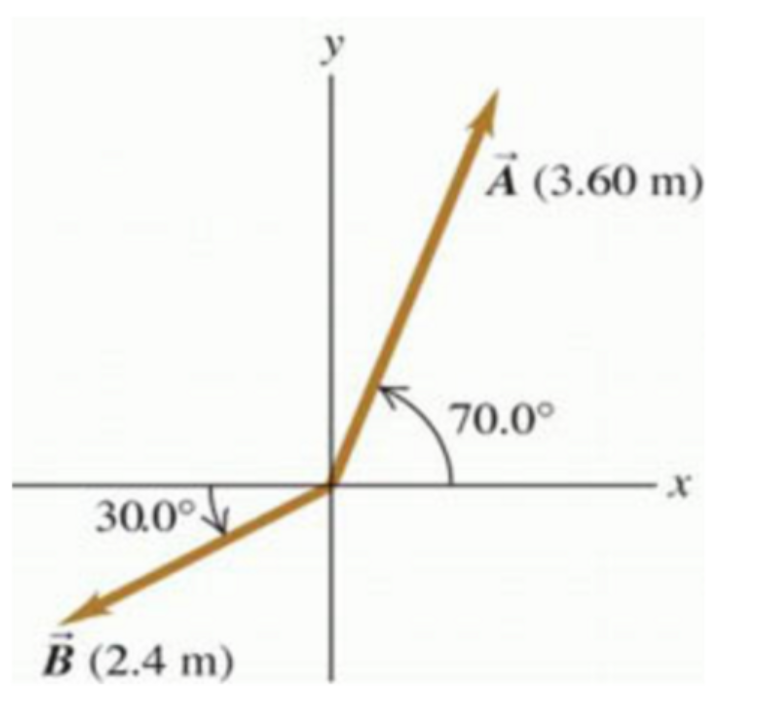# Problem: a) For the two vectors A?  and B?  in the figure (Figure 1) , find the scalar product A?B? b) Find the magnitude of the vector product AxB

###### FREE Expert Solution

Scalar product:

$\overline{){\mathbf{A}}{\mathbf{•}}{\mathbf{B}}{\mathbf{=}}{\mathbf{A}}{\mathbf{B}}{\mathbf{c}}{\mathbf{o}}{\mathbf{s}}{\mathbf{\theta }}}$

Cross product:

Magnitude:

$\overline{)|\stackrel{⇀}{\mathbit{A}}|{=}\sqrt{{{\mathbit{A}}_{\mathbit{x}}}^{2}+{{\mathbit{A}}_{\mathbit{y}}}^{2}+{{\mathbit{A}}_{\mathbit{z}}}^{2}}}$

a)

The angle between A and B = (180 - 70.0°) + 30.0° = 140.0°

87% (155 ratings)###### Problem Details

a) For the two vectors A?  and B?  in the figure (Figure 1) , find the scalar product A?B

b) Find the magnitude of the vector product AxBFrequently Asked Questions

What scientific concept do you need to know in order to solve this problem?

Our tutors have indicated that to solve this problem you will need to apply the Intro to Cross Product (Vector Product) concept. If you need more Intro to Cross Product (Vector Product) practice, you can also practice Intro to Cross Product (Vector Product) practice problems.

What professor is this problem relevant for?

Based on our data, we think this problem is relevant for Professor Shindler's class at MSU.